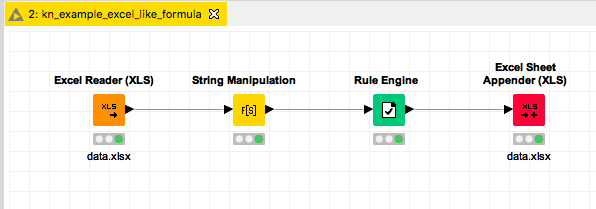# Excel like formulas on Strings

Hi,
I want to add some columns with calculations or IF formulas.

I tried the math node, but I cannot select the columns I want, because they don’t contain numbers. In excel you can use some formulas on text based columns.
I tried the rule engine, but I can’t add formulas in the rules.

Example of what I want to do:

Data:
OBE10
OBE11
PBE20
PBE21

If the cell in the column Data begins with the letter O, write the value of the cell, so OBE10 in the first row. If the cell begins with P, write “referral to another column”. So the function LEFT in combination with an IF formula.

The total formula is a bit more difficult, but if I know how I can add formulas on text, I can continue.
In excel, it would look something like:
=IF(AND(LEFT(AL2,1)=“O”,LEFT(A2,1)=“P”,OR(B2=“x”,B2=“y”)),AL2,A2)
Kind regards,
Tienemientje

I do have a solution by adding extra nodes, like string manipulation to add an extra column for the first letter. But I would like to know if there is a better solution.
Kind regards, Tienemientje

One option would be to combine String Manipulation and Rule Engine as quite powerful tools. Another possibility would be to do all that in a Java Snippet. The two mentioned nodes make the functions more accessible.kn_example_excel_like_formula.knwf (24.2 KB)

1 Like

Thank you very much @mlauber71 The string manipulation and rule engine were indeed what I also found. I’m a newbie and looking into the program and sometimes I think I do a lot of unneeded steps to get to a result.
But even if I have a screen full of nodes, I really like the program, in the end it will save me a lot of time running these reports every time. So I should step away a bit of my excel thinking and trying to combine everything to reduce the number of columns.

Kind regards and another big thank you, Tienemientje

2 Likes

With our upcoming release, which will be online very soon, we have a new node which can be used for those formulas.

5 Likes

Excel is a great tool which I use a lot. Also you could do a lot of things in R or Python (and would not need KNIME). And yes often KNIME uses a lot of nodes to get a result that you otherwise might get with a few lines of code.

The major benefit of KNIME is you could bring all that together and have a graphical interface that can easily reproduce your procedures and you can see how the nodes all work together. You might also add comments and use MetaNodes to structure your workflows. And with the traffic sign logic you would get a feedback if your workflow is working and where there might be technical/logical problems.

The ‘philosophy’ of KNIME imho is very well captured in this article:

Give a lot of people easy access to data analytics tools and strike a balance between advanced coding and easy usage.

String temp_value = “”;

if (c_data_value == null) {temp_value = “”;}
else if(c_data_value.trim().substring(0, 1).equals(“O”)) {temp_value = c_data_value;}
else if(c_data_value.trim().substring(0, 1).equals(“P”)) {temp_value = c_alternative_value;}
else {temp_value = “something else”;}

out_result = temp_value;
out_test_output = c_data_value.trim().substring(0, 1);

kn_example_excel_like_formula.knwf (28.3 KB)

1 Like

Hi Iris, can you tell us which is the node you mention? It could be very useful in ‘simple’ manipulatiions.
thanks a lot

it is the Column Expression node.
You need to install it from the labs update site.

https://hub.knime.com/knime/nodes/Column_Expressions*2_0ji_xeG-SudFu-

Best, Iris

2 Likes

Great!! thanks